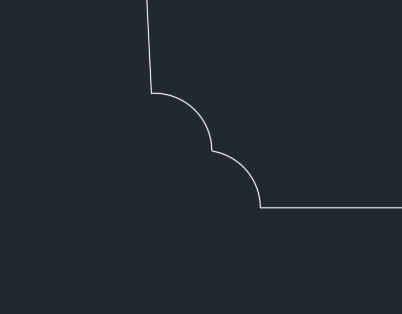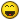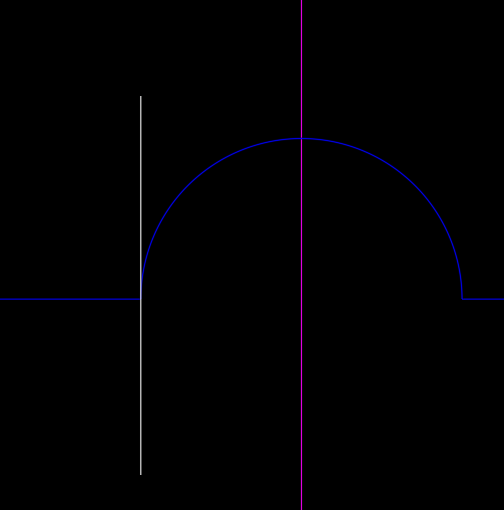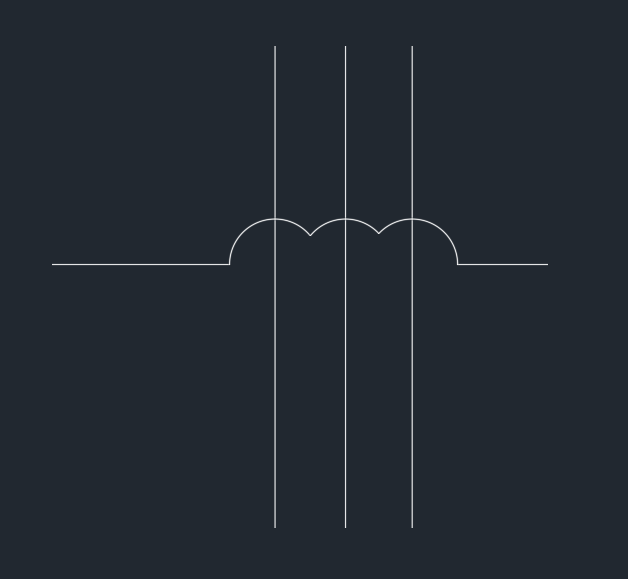# Connect lines connected with an arc## Recommended PostsHi fellow coders,

This is the biggest request that I will be making in this forum to this date. I don't really expect any solutions or codes, any ideas are also welcomed and I can try to do them once I've heard your opinions.

Here's the story: There are some lines drawn (as shown on the left) which represent pex connecting to different fixtures in an apartment. My colleagues will design this, and then produce the drawings on the right (using the attached LISP code that I created and provide them). I can never have access to the design on the left unless my colleagues issue the final drawing up for construction so that I can start detailing. Therefore I will always get the drawings on the right. Now I'm trying to find the easiest and quickest way to reverse this process, but to no luck. I welcome some ideas you might have (except asking my colleague not to do this). [Now the LISP routine might not be perfect because it does produce unexpected results when done on polylines near the vertices, but I highly doubt this will ever be the case. If there are polylines, there will never be any arc segments on it.]

This is the worst case scenario, but I don't think it will ever get to this point.Thanks,

Jonathan Handojo

##### Share on other sitesAs there arcs you know start end points do a little sq aroud the point use ssget "CP" it will find the two objects connected in dwg they are a line ok ake a new line pt1 pt2 etc then pedit entlast join ent1 ent2. This will give a 3 segment pline.

Version 2 ok again a little sq around end pts find 2 lines do a check and work out extreme away points p3 p4 erase 2 lines and arc draw a new line  p3 -p4

Edited by BIGAL
##### Share on other sitesTheres a routine on internet to join collinear lines which was done by Lee Mac. Would serve a good purpose here.

Here is my attempt (which simply erases the ARC entites, creates lines and implements his 'Join colinear lines' by layer):

```; https://forums.autodesk.com/t5/visual-lisp-autolisp-and-general/join-lines-by-layer/td-p/7051838
; Join Lines  -  Lee Mac

; Modification of 'Join Lines'
(defun C:test ( / groupbykey LM:Collinear-p process _Line _GetEnds )

; LM
; http://www.theswamp.org/index.php?topic=53515.0
(defun groupbykey ( lst / rtn tmp )
(foreach itm (reverse lst)
(if (setq tmp (assoc (car itm) rtn))
(setq rtn (subst (vl-list* (car itm) (cdr itm) (cdr tmp)) tmp rtn))
(setq rtn (cons  (list (car itm) (cdr itm)) rtn))
)
)
)

;; Collinear-p  -  Lee Mac
;; Returns T if p1,p2,p3 are collinear
(defun LM:Collinear-p ( p1 p2 p3 )
(
(lambda ( a b c )
(or
(equal (+ a b) c 1e-1)
(equal (+ b c) a 1e-1)
(equal (+ c a) b 1e-1)
)
)
(distance p1 p2) (distance p2 p3) (distance p1 p3)
)
)

; LM's Sub from his 'Join Lines' Routine
(defun process ( l / x r )
(if (setq x (car l))
(progn
(foreach y (cdr l)
(if (vl-every '(lambda ( a ) (apply 'LM:collinear-p (cons a (cdr x)))) (cdr y))
(setq x (cons (car x) (LM:furthestapart (append (cdr x) (cdr y)))))
(setq r (cons y r))
)
)
(entmake (append (car x) (mapcar 'cons '(10 11) (cdr x))))
(process r)
)
)
)

(defun _Line (lyr p1 p2)
(entmakex (list (cons 0 "LINE")(cons 8 lyr) (cons 10 p1)(cons 11 p2)))
)

(defun _GetEnds ( e )
(mapcar '(lambda (x) (vlax-curve-getPointAtParam e x))
(list (vlax-curve-getStartParam e) (vlax-curve-getEndParam e))
)
)

( ; Main
(lambda ( /   SS arcsL linesL )

(cond
( (not (setq SS (ssget '((0 . "LINE,ARC"))))) )
(
(
(lambda ( SS / i enx e lyr tmp )
(repeat (setq i (sslength SS))
(setq enx (entget (setq e (ssname SS (setq i (1- i))))))
(setq lyr (cdr (assoc 8 enx)))
(cond
( (member '(0 . "LINE") enx)
(setq linesL (cons (list lyr (list enx (cdr (assoc 10 enx)) (cdr (assoc 11 enx)))) linesL))
(entdel e)
)
( (member '(0 . "ARC") enx)
(setq linesL (cons (list lyr (list (setq tmp (entget (apply '_Line (cons lyr (_GetEnds e))))) (cdr (assoc 10 tmp)) (cdr (assoc 11 tmp)) ) ) linesL))
(entdel (cdr (assoc -1 tmp)))
(entdel e)
)
); cond
); repeat
nil
); lambda
SS
)
)
(t
(foreach x (groupbykey linesL)
(process (apply 'append (cdr x)))
); foreach
); t
); cond
); lambda
)
(princ)
); defun
```

Although it won't deal with your worst case scenario, I think its possible to do it via Lee's Chain Selection algorithm, to determine the 'vectors' of the touching lines with arcs and calculate the potential intersection (when they are not collinear).

I might sound like a math guy here, but trust me I'm not.Oh, yea and sorry for my sloppy coding.

Edited by Grrr
##### Share on other sites@Grrr Thanks for the idea. It certainly makes sense. I had an idea somewhere along the lines of how you did your codes too, but then you'll have to check on discontinuity of the lines too. Supposedly you copy the design on the right and place it somewhere on the right horizontally like so (or mirrored): (and surprisingly vertically as well).

Even though the lines from the separate design are not connected, they are still collinear. And even if it's not a separate design in another room, it could be one design in the same room where two unconnected collinear lines could exist.

@BIGAL The closest I've gotten was your idea. I don't think this will happen, but your idea would fail if some other existing lines touches the arcs like this. Since it's a shop drawing, there's way more elements in it than just a few simple lines. Then ssget will return multiple curves, and you won't know which curve to retrieve. (Well, I mean my LISP routine does match the properties of the arc to the curve, so I guess I can put some filters into the ssget to make it more robust, so your idea would be worth a shot.):I appreciate your opinions guys! Thanks!

##### Share on other sitesAny way to get them to use polylines to draft? I have this code HERE that might help the 'hop' process. Of course it needs to be adapted for layers .. right now just does all intersections.

*Edit .. here's an idea ( maybe not a good one) .. store these lines as xdata and recreate them later ?

Edited by ronjonp
##### Share on other sitesAs your looking for arcs can match layer when looking for the line next to it, as you say depends on a true dwg. The other thing is if you look at the line angle of the two arc points and compare the line angle with say a Equal can have a tolerance again using a layer filter also would help. need maybe a  = ang or = ang + Pi

##### Share on other sites18 hours ago, Jonathan Handojo said:

but then you'll have to check on discontinuity of the lines too. Supposedly you copy the design on the right and place it somewhere on the right horizontally like so (or mirrored): (and surprisingly vertically as well)

Thats why you must rely on the scope of the selection you do with (ssget "_:L-I").

In my own LISP routines that I use and which I wrote myself: I avoid purposely this (ssget "_X") automation,

just because one day I could forget the algorithm I used there and mess up stuff in my drawing, similarly to your case.

Although I remember somewhere on the forums was written routine for multiple bounding boxes for multiple 'selections', which could potentially differentiate your designs.

HTH##### Share on other sites@Jonathan Handojo  Do these under/over rules need to be determined one pick at a time? Can they be determined by layers?

I do irrigation piping and hops denote that the pipes are not connected and we control this by layer ... here's something to mull over if this could be the case for you too```(defun c:hops (/ a b d e ee i p p1 p2 pts s x)
;; RJP » 2019-01-21
;; Creates hops on a selection of intersecting polylines
;; Will not create hops on objects that share the same layer
(setq a 100)
(cond
((and (setq s (ssget ":L" '((0 . "lwpolyline"))))
(> (sslength s) 1)
(setq s (mapcar 'vlax-ename->vla-object (vl-remove-if 'listp (mapcar 'cadr (ssnamex s)))))
(setq s (mapcar '(lambda (x) (cons x (vla-get-layer x))) s))
(setq s (vl-sort s '(lambda (r j) (> (cdr r) (cdr j)))))
(setq b s)
)
(foreach e	s
(setq ee (car e))
(if (setq pts (apply 'append
(mapcar '(lambda (x)
(if (/= (cdr e) (cdr x))
(vlax-invoke ee 'intersectwith (car x) 0)
)
)
(setq b (cdr b))
)
)
)
(cond ((and (setq p (list (car pts) (cadr pts)))
(setq i (vlax-curve-getparamatpoint ee p))
(= 0.0 (vla-getbulge ee (fix i)))
(setq d (vlax-curve-getdistatpoint ee p))
(setq p1 (vlax-curve-getpointatdist ee (+ d a)))
(setq p2 (vlax-curve-getpointatdist ee (- d a)))
)
(vlax-invoke ee 'addvertex (setq i (1+ (fix i))) (mapcar '+ p1 '(0 0)))
(vlax-invoke ee 'addvertex i (mapcar '+ p2 '(0 0)))
(vla-setbulge
ee
i
(cond ((< (angle '(0 0) p1) (angle '(0 0) p2)) -1)
(1)
)
)
)
)
(setq pts (cdddr pts))
)
)
)
)
)
(princ)
)
;; Convert lines to polylines
(defun c:c-l (/ line->polyline ss)
(defun line->polyline	(ename / el)
(if	(setq el (entget ename '("*")))
(progn (entdel ename)
(entmakex (mapcar '(lambda	(x)
(cond	((= (car x) 0) '(0 . "LWPOLYLINE"))
((= (cdr x) "AcDbLine") '(100 . "AcDbPolyline"))
((= (car x) 11) (cons 10 (cdr x)))
(x)
)
)
(append (reverse (member '(100 . "AcDbLine") (reverse el)))
'((90 . 2) (43 . 0.0) (38 . 0.0) (70 . 0))
(cdr (member '(100 . "AcDbLine") el))
)
)
)
)
)
)
(if (setq ss (ssget ":L" '((0 . "LINE"))))
(foreach l (vl-remove-if 'listp (mapcar 'cadr (ssnamex ss))) (line->polyline l))
)
)```

##### Share on other sitesOh, I should mention (and I forgot to do so in the OP) that this does tend to happen quite some often. But never on the polyline vertex where it's not collinear like I showed on the worst case scenario. The LISP code that I attached is able to catch this.@ronjonp I would suspect that all the lines on one layer will be below the other. Which would add a better purpose. However, I can't guarantee that it will always work this way.

Also, you're right. They would do their shop drawings on their own layers. Afterwards, I select those curves and copy them to my own layers. To do this, I have other LISP codes, but I won't be sharing them here unless probably otherwise. The layers I create come from the below (which is part of what you see from the attached dwg file):

```(mapcar '(lambda (x) (apply 'strcat x)) (JH:listcombination '(("RoughIn ") ("Hot " "Cold " "Warm ") ("16" "20" "25") ("mm"))))

;; JH:listcombination --> Jonathan Handojo
;; Returns all possible combinations of each element from an association list
;; lst - association list (or list of lists)
;; Example call:
;; _\$ (JH:listcombination '(("A" "B") ("C" "D") ("E" "F")))
;; (("A" "C" "E") ("A" "C" "F") ("A" "D" "E") ("A" "D" "F") ("B" "C" "E") ("B" "C" "F") ("B" "D" "E") ("B" "D" "F"))

(defun JH:listcombination (lst)
(if (null lst) '(nil)
(apply 'append
(mapcar
'(lambda (x)
(mapcar
'(lambda (y)
(cons x y)
)
(JH:listcombination (cdr lst))
)
)
(car lst)
)
)
)
)```

Edited by Jonathan Handojo
##### Share on other sites3 arcs interesting again though the ssget CP using a small box will find the next arc so is it arc or line ? If so look again for next arc and repeat is arc or line. Will play later today it would be a automated thing do all will start with 1 arc. Then think about 2 or more.

## Join the conversation

You can post now and register later. If you have an account, sign in now to post with your account.
Note: Your post will require moderator approval before it will be visible.×   Pasted as rich text.   Restore formatting

Only 75 emoji are allowed.